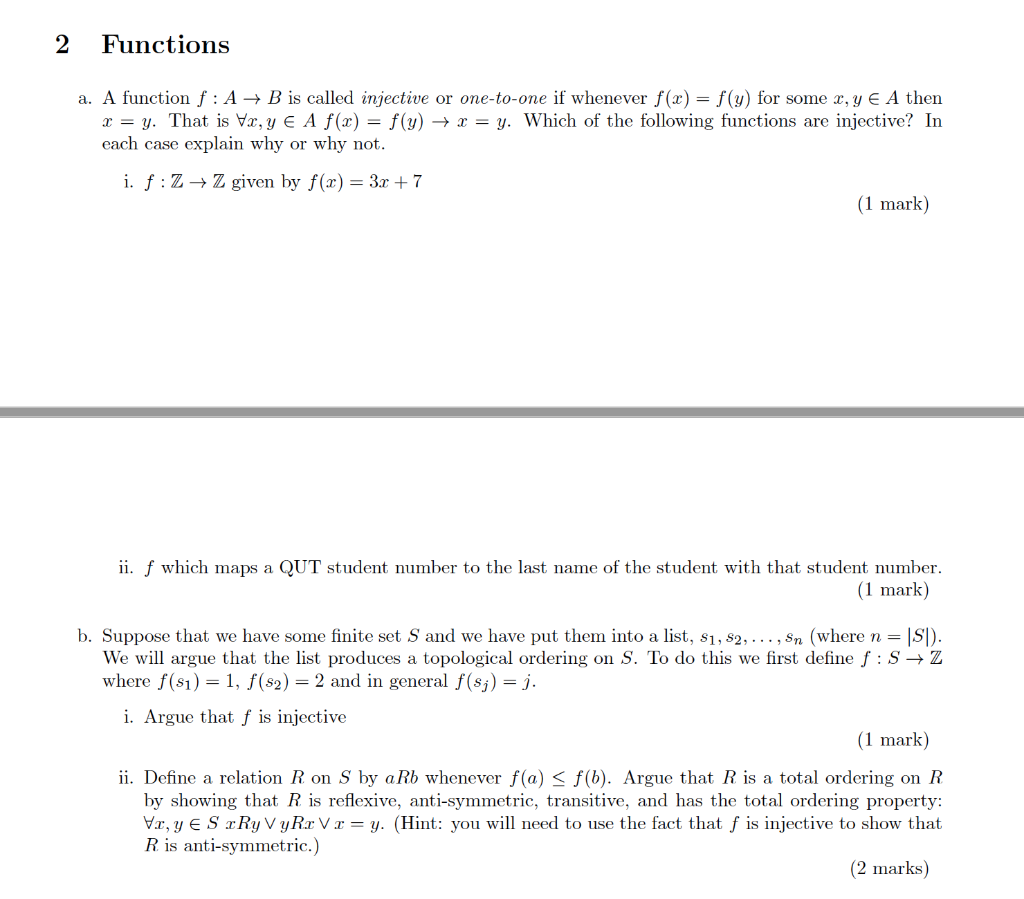# 2 Functions a. A function f : A-B is called injective or one-to-one if whenever f(x)-f(y) for some x, y

###### Question:2 Functions a. A function f : A-B is called injective or one-to-one if whenever f(x)-f(y) for some x, y E A then x = y. That is Vz, y A f(x) = f(y) → x = y. Which of the following functions are injective? In each case explain why or why not i. f:Z-Z given by f() 3r +7 (1 mark ii. f which maps a QUT student number to the last name of the student with that student number 1 mark b. Suppose that we have some finite set S and we have put thern into a list, 31, s2, . . . , sn (where n = ISI) We will argue that the list produces a topological ordering on S. To do this we first define f:sZ where f(s1)-1, f(s2)2 and in general f(sj)-j i. Argue that f is injective 1 mark ii. Define a relation R on S by aRb whenever f(a) S f(b). Argue that R is a total ordering on R by showing that R is reflexive, anti-symmetric, transitive, and has the total ordering property: Vz, y E S rRyVyRr Vr = y. (Hint: you will need to use the fact that f is injective to show that R is anti-symmetric.) (2 marks)

#### Similar Solved Questions

##### 5. Radix Sort Sort the following array using a radix sort with base 10, showing each...
5. Radix Sort Sort the following array using a radix sort with base 10, showing each pass a. .791316.64|.39|.20|.89.53|.71.42...
##### Data collected Cu(NO3)2 | Zn(NO3)2 = 0.999 V Pb | 1.0 M Pb(NO3)2 || 1.0 M...
data collected Cu(NO3)2 | Zn(NO3)2 = 0.999 V Pb | 1.0 M Pb(NO3)2 || 1.0 M Zn(NO3)2 | Zn = 0.396 V PART B: REDUCTION POTENTIALS 1. Report the measured cell potential for each galvanic cell and state which electrode corresponds to the cathode and which to the anode. 2. Given E = -0.76 V for the Zn/Z...
##### П. Akimora Dairy began operations on April 1, 2015, with purchase of 250 milking cows for...
П. Akimora Dairy began operations on April 1, 2015, with purchase of 250 milking cows for ¥8,500,000. It has completed the first month of operations and has the following information for its milking cows at the end of April 2015 (000 omitted). Milking cows ¥(250,000) Change in fair val...